# RS Aggarwal Solutions for Class 7 Maths Exercise 8A Chapter 8 Ratio and Proportion

RS Aggarwal Solutions for Class 7 Maths Exercise 8A Chapter 8 Ratio and Proportion in simple PDF are given here. This exercise of RS Aggarwal Solutions for Class 7 Chapter 8 contains questions related to the ratio in simplest form and comparison of ratios. The ratio of two quantities of the same kind and in the same units is the fraction that one quantity is of others. The ratio a is to b is the fraction (a/b), written as a : b. Learn more about these topics by solving the questions of RS Aggarwal Solutions for Class 7 Maths Chapter 8 Ratio and Proportion with the help of solutions provided here.

## Download the PDF of RS Aggarwal Solutions For Class 7 Maths Chapter 8 Ratio and Proportion – Exercise 8A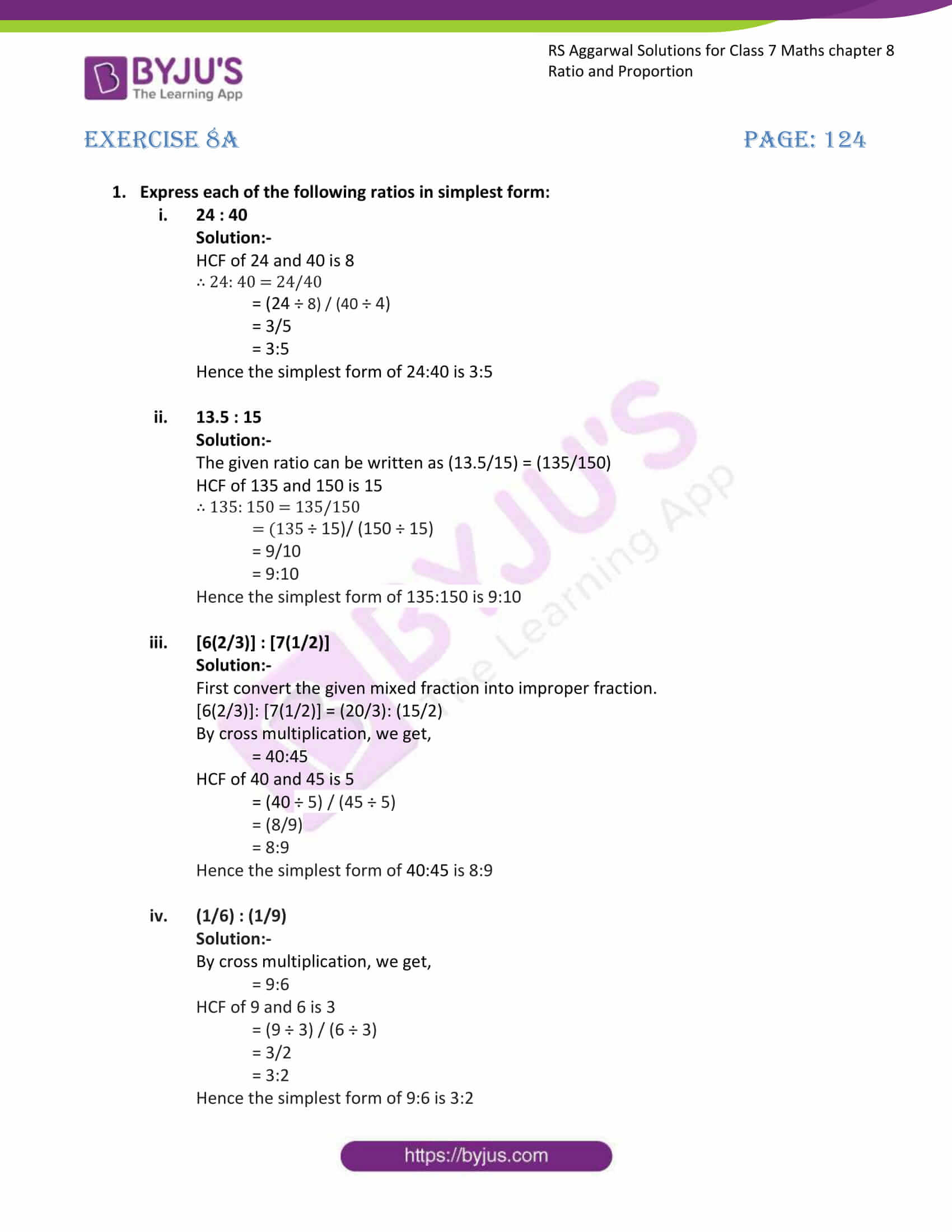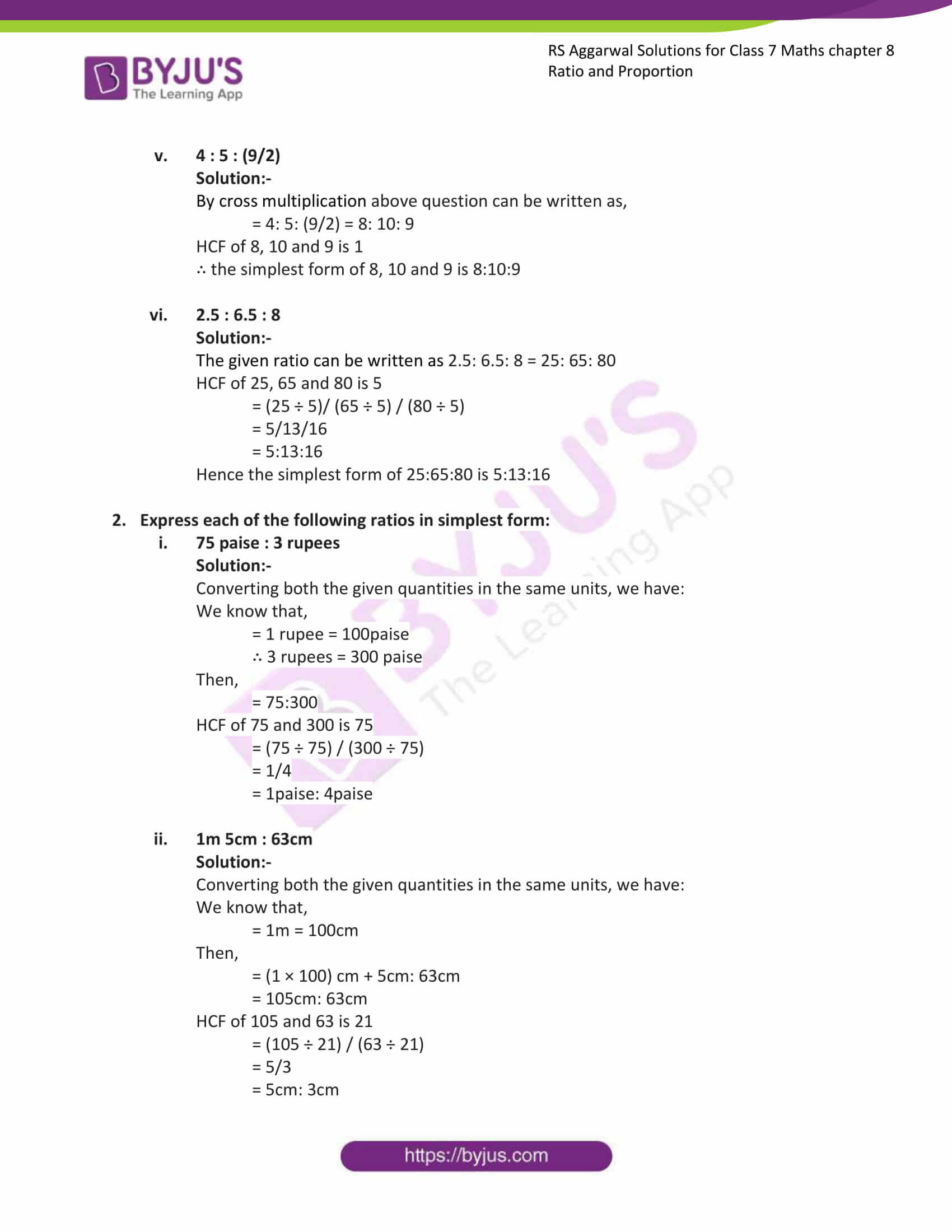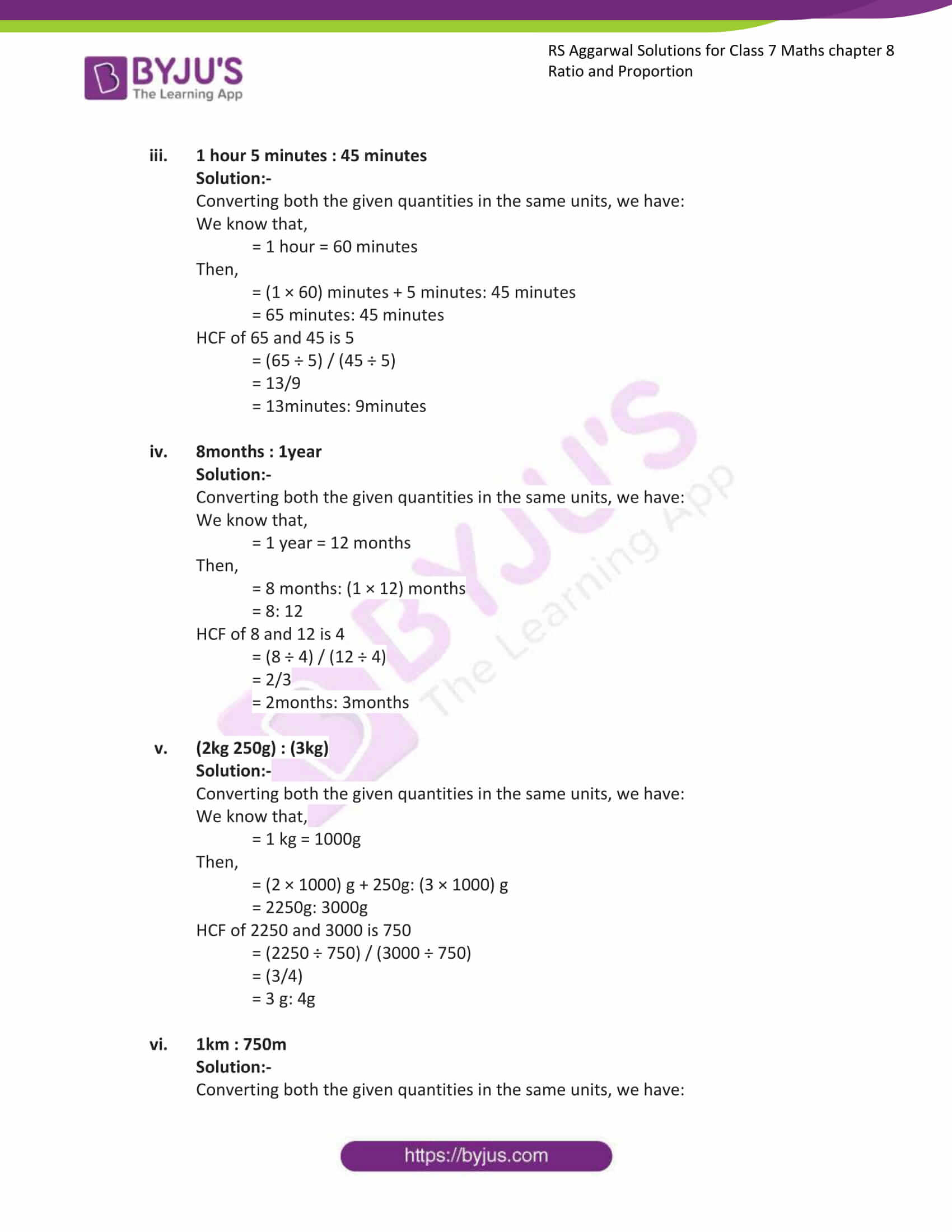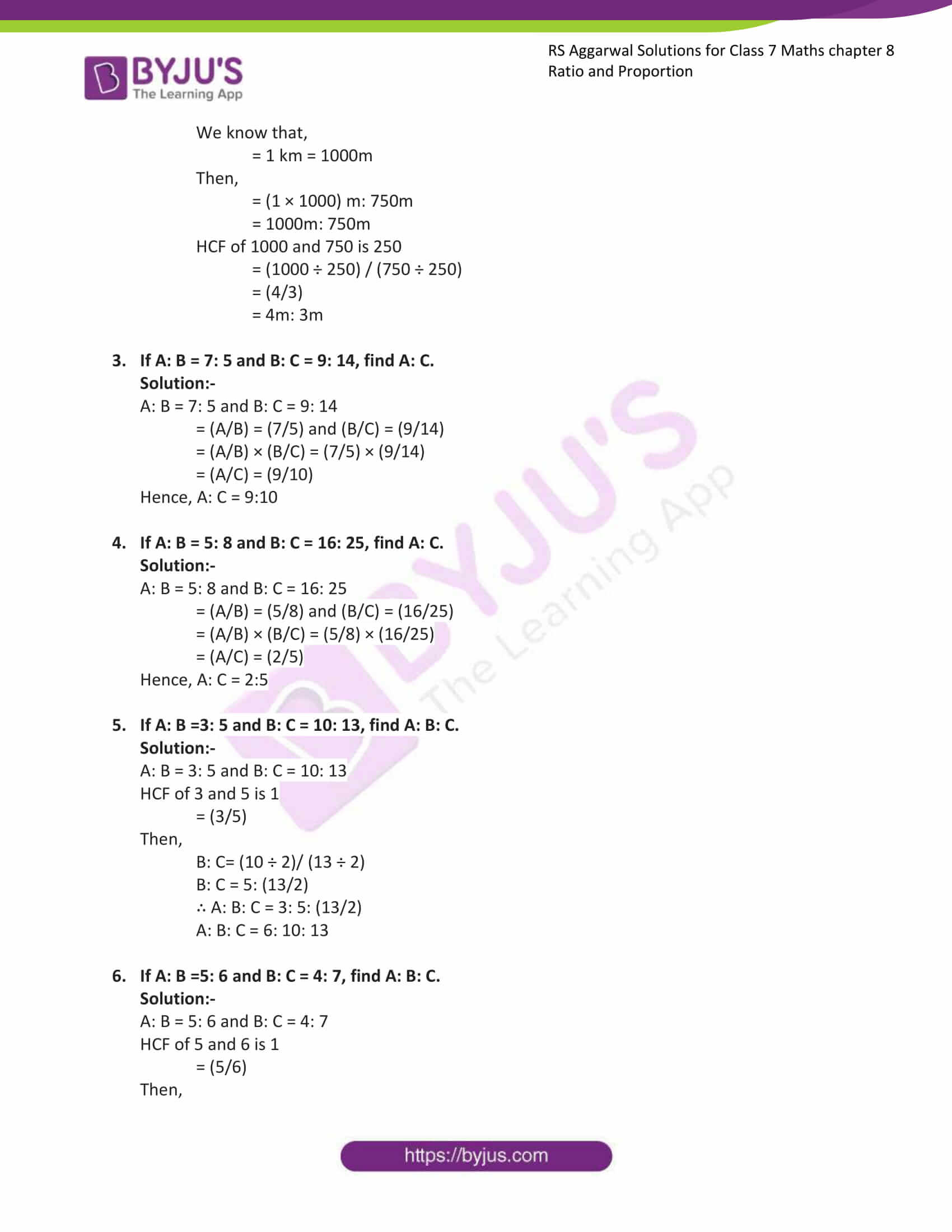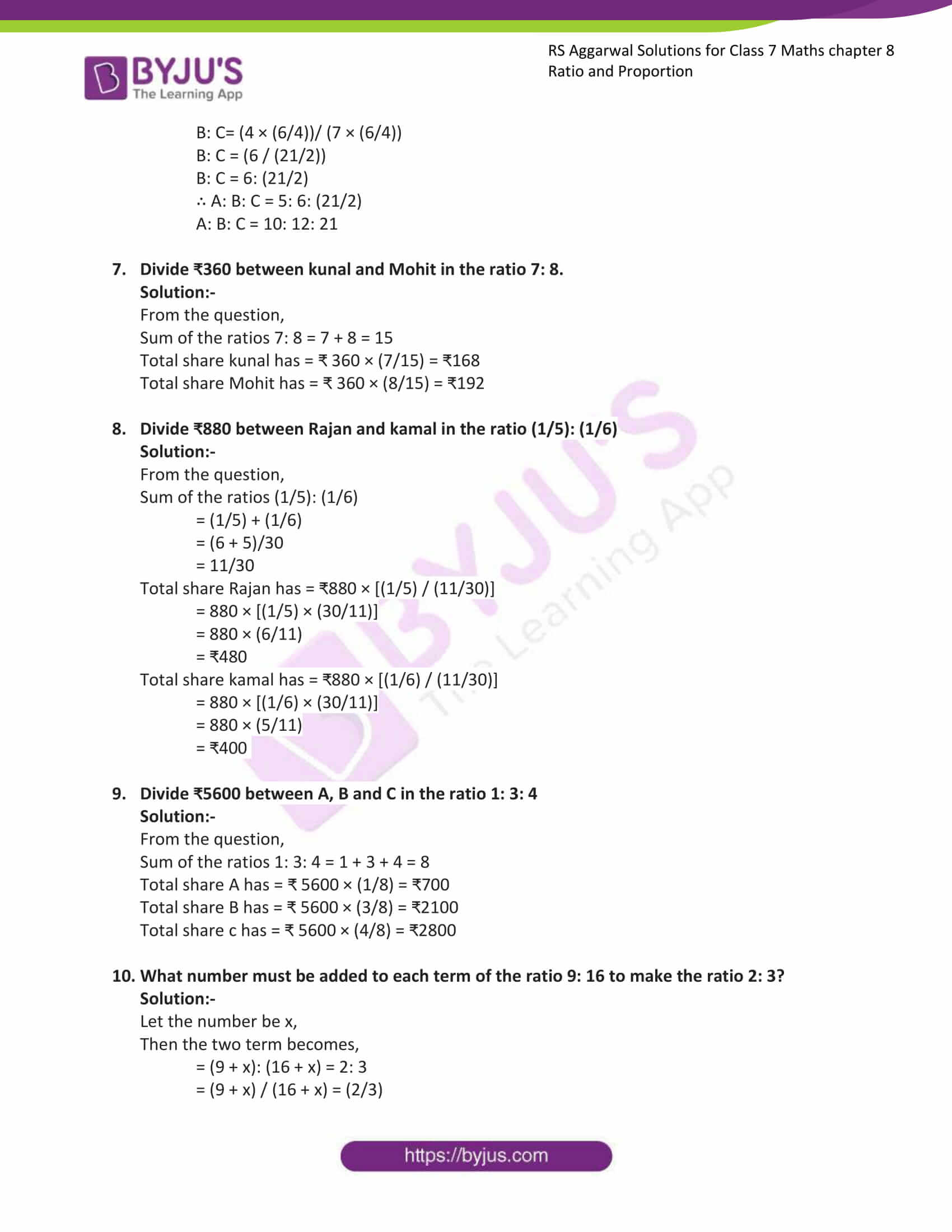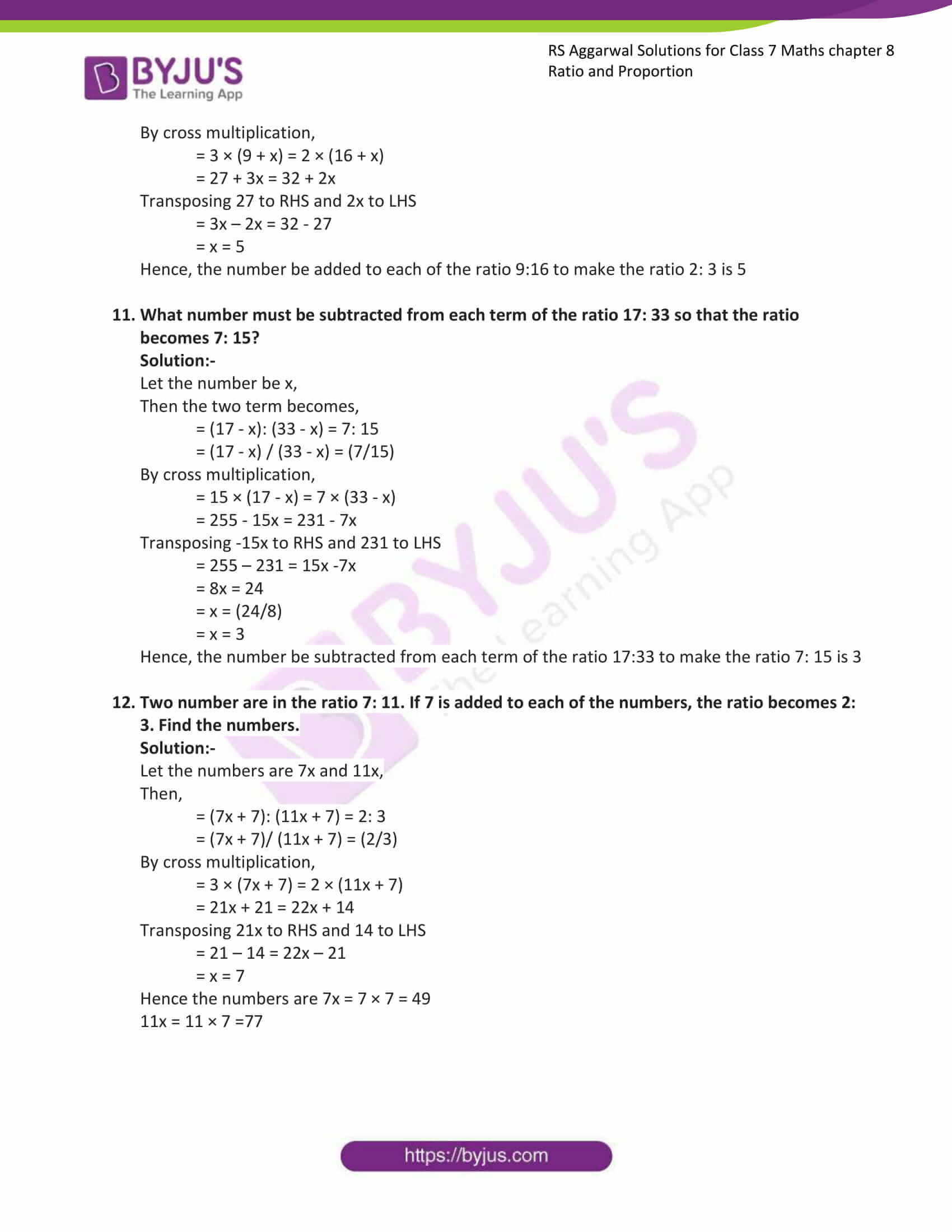### Access answers to Maths RS Aggarwal Solutions for Class 7 Chapter 8 – Ratio and Proportion Exercise 8A

1. Express each of the following ratios in simplest form:

1. 24 : 40

Solution:-

HCF of 24 and 40 is 8

∴ 24: 40 = 24/40

= (24 ÷ 8) / (40 ÷ 4)

= 3/5

= 3:5

Hence the simplest form of 24:40 is 3:5

2. 13.5 : 15

Solution:-

The given ratio can be written as (13.5/15) = (135/150)

HCF of 135 and 150 is 15

∴ 135: 150 = 135/150

= (135 ÷ 15)/ (150 ÷ 15)

= 9/10

= 9:10

Hence the simplest form of 135:150 is 9:10

3. [6(2/3)] : [7(1/2)]

Solution:-

First convert the given mixed fraction into improper fraction.

[6(2/3)]: [7(1/2)] = (20/3): (15/2)

By cross multiplication, we get,

= 40:45

HCF of 40 and 45 is 5

= (40 ÷ 5) / (45 ÷ 5)

= (8/9)

= 8:9

Hence the simplest form of 40:45 is 8:9

4. (1/6) : (1/9)

Solution:-

By cross multiplication, we get,

= 9:6

HCF of 9 and 6 is 3

= (9 ÷ 3) / (6 ÷ 3)

= 3/2

= 3:2

Hence the simplest form of 9:6 is 3:2

5. 4 : 5 : (9/2)

Solution:-

By cross multiplication above question can be written as,

= 4: 5: (9/2) = 8: 10: 9

HCF of 8, 10 and 9 is 1

∴ the simplest form of 8, 10 and 9 is 8:10:9

6. 2.5 : 6.5 : 8

Solution:-

The given ratio can be written as 2.5: 6.5: 8 = 25: 65: 80

HCF of 25, 65 and 80 is 5

= (25 ÷ 5)/ (65 ÷ 5) / (80 ÷ 5)

= 5/13/16

= 5:13:16

Hence the simplest form of 25:65:80 is 5:13:16

2. Express each of the following ratios in simplest form:

1. 75 paise : 3 rupees

Solution:-

Converting both the given quantities in the same units, we have:

We know that,

= 1 rupee = 100paise

∴ 3 rupees = 300 paise

Then,

= 75:300

HCF of 75 and 300 is 75

= (75 ÷ 75) / (300 ÷ 75)

= 1/4

= 1paise: 4paise

2. 1m 5cm : 63cm

Solution:-

Converting both the given quantities in the same units, we have:

We know that,

= 1m = 100cm

Then,

= (1 × 100) cm + 5cm: 63cm

= 105cm: 63cm

HCF of 105 and 63 is 21

= (105 ÷ 21) / (63 ÷ 21)

= 5/3

= 5cm: 3cm

3. 1 hour 5 minutes : 45 minutes

Solution:-

Converting both the given quantities in the same units, we have:

We know that,

= 1 hour = 60 minutes

Then,

= (1 × 60) minutes + 5 minutes: 45 minutes

= 65 minutes: 45 minutes

HCF of 65 and 45 is 5

= (65 ÷ 5) / (45 ÷ 5)

= 13/9

= 13minutes: 9minutes

4. 8months : 1year

Solution:-

Converting both the given quantities in the same units, we have:

We know that,

= 1 year = 12 months

Then,

= 8 months: (1 × 12) months

= 8: 12

HCF of 8 and 12 is 4

= (8 ÷ 4) / (12 ÷ 4)

= 2/3

= 2months: 3months

5. (2kg 250g) : (3kg)

Solution:-

Converting both the given quantities in the same units, we have:

We know that,

= 1 kg = 1000g

Then,

= (2 × 1000) g + 250g: (3 × 1000) g

= 2250g: 3000g

HCF of 2250 and 3000 is 750

= (2250 ÷ 750) / (3000 ÷ 750)

= (3/4)

= 3 g: 4g

6. 1km : 750m

Solution:-

Converting both the given quantities in the same units, we have:

We know that,

= 1 km = 1000m

Then,

= (1 × 1000) m: 750m

= 1000m: 750m

HCF of 1000 and 750 is 250

= (1000 ÷ 250) / (750 ÷ 250)

= (4/3)

= 4m: 3m

3. If A: B = 7: 5 and B: C = 9: 14, find A: C.

Solution:-

A: B = 7: 5 and B: C = 9: 14

= (A/B) = (7/5) and (B/C) = (9/14)

= (A/B) × (B/C) = (7/5) × (9/14)

= (A/C) = (9/10)

Hence, A: C = 9:10

4. If A: B = 5: 8 and B: C = 16: 25, find A: C.

Solution:-

A: B = 5: 8 and B: C = 16: 25

= (A/B) = (5/8) and (B/C) = (16/25)

= (A/B) × (B/C) = (5/8) × (16/25)

= (A/C) = (2/5)

Hence, A: C = 2:5

5. If A: B =3: 5 and B: C = 10: 13, find A: B: C.

Solution:-

A: B = 3: 5 and B: C = 10: 13

HCF of 3 and 5 is 1

= (3/5)

Then,

B: C= (10 ÷ 2)/ (13 ÷ 2)

B: C = 5: (13/2)

∴ A: B: C = 3: 5: (13/2)

A: B: C = 6: 10: 13

6. If A: B =5: 6 and B: C = 4: 7, find A: B: C.

Solution:-

A: B = 5: 6 and B: C = 4: 7

HCF of 5 and 6 is 1

= (5/6)

Then,

B: C= (4 × (6/4))/ (7 × (6/4))

B: C = (6 / (21/2))

B: C = 6: (21/2)

∴ A: B: C = 5: 6: (21/2)

A: B: C = 10: 12: 21

7. Divide ₹360 between kunal and Mohit in the ratio 7: 8.

Solution:-

From the question,

Sum of the ratios 7: 8 = 7 + 8 = 15

Total share kunal has = ₹ 360 × (7/15) = ₹168

Total share Mohit has = ₹ 360 × (8/15) = ₹192

8. Divide ₹880 between Rajan and kamal in the ratio (1/5): (1/6)

Solution:-

From the question,

Sum of the ratios (1/5): (1/6)

= (1/5) + (1/6)

= (6 + 5)/30

= 11/30

Total share Rajan has = ₹880 × [(1/5) / (11/30)]

= 880 × [(1/5) × (30/11)]

= 880 × (6/11)

= ₹480

Total share kamal has = ₹880 × [(1/6) / (11/30)]

= 880 × [(1/6) × (30/11)]

= 880 × (5/11)

= ₹400

9. Divide ₹5600 between A, B and C in the ratio 1: 3: 4

Solution:-

From the question,

Sum of the ratios 1: 3: 4 = 1 + 3 + 4 = 8

Total share A has = ₹ 5600 × (1/8) = ₹700

Total share B has = ₹ 5600 × (3/8) = ₹2100

Total share c has = ₹ 5600 × (4/8) = ₹2800

10. What number must be added to each term of the ratio 9: 16 to make the ratio 2: 3?

Solution:-

Let the number be x,

Then the two term becomes,

= (9 + x): (16 + x) = 2: 3

= (9 + x) / (16 + x) = (2/3)

By cross multiplication,

= 3 × (9 + x) = 2 × (16 + x)

= 27 + 3x = 32 + 2x

Transposing 27 to RHS and 2x to LHS

= 3x – 2x = 32 – 27

= x = 5

Hence, the number be added to each of the ratio 9:16 to make the ratio 2: 3 is 5

11. What number must be subtracted from each term of the ratio 17: 33 so that the ratio becomes 7: 15?

Solution:-

Let the number be x,

Then the two term becomes,

= (17 – x): (33 – x) = 7: 15

= (17 – x) / (33 – x) = (7/15)

By cross multiplication,

= 15 × (17 – x) = 7 × (33 – x)

= 255 – 15x = 231 – 7x

Transposing -15x to RHS and 231 to LHS

= 255 – 231 = 15x -7x

= 8x = 24

= x = (24/8)

= x = 3

Hence, the number be subtracted from each term of the ratio 17:33 to make the ratio 7: 15 is 3

12. Two number are in the ratio 7: 11. If 7 is added to each of the numbers, the ratio becomes 2: 3. Find the numbers.

Solution:-

Let the numbers are 7x and 11x,

Then,

= (7x + 7): (11x + 7) = 2: 3

= (7x + 7)/ (11x + 7) = (2/3)

By cross multiplication,

= 3 × (7x + 7) = 2 × (11x + 7)

= 21x + 21 = 22x + 14

Transposing 21x to RHS and 14 to LHS

= 21 – 14 = 22x – 21

= x = 7

Hence the numbers are 7x = 7 × 7 = 49

11x = 11 × 7 =77

### Access other exercises of RS Aggarwal Solutions For Class 7 Chapter 8 – Ratio and Proportion

Exercise 8C Solutions